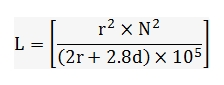# Spiral Coil Inductance Calculator

#### Resultwhere,

L = Inductance

r = Coil Mean Radius

N = Wire Coil Number of Turns

d = Coil Depth